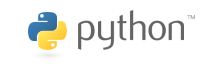# Data analysis: Pandas and Seaborn¶

## Yoav Ram¶Pandas is a very strong library for manipulating large and complex datasets using a new data structure, the data frame, which models a table of data. Pandas helps to close the gap between Python and R for data analysis and statistical computing.

Pandas data frames address three deficiencies of NumPy arrays:

• data frame hold heterogenous data; each column can have its own numpy.dtype,
• the axes of a data frame are labeled with column names and row indices,
• and, they account for missing values which this is not directly supported by arrays.

Data frames are extremely useful for data manipulation. They provide a large range of operations such as filter, join, and group-by aggregation, as well as plotting.

In :
import pandas as pd
print('Pandas version:', pd.__version__)

Pandas version: 0.24.2


# Statistical Analysis of Life History Traits¶

We will analyze animal life-history data from AnAge.

In :
data = pd.read_csv('../data/anage_data.txt', sep='\t') # lots of other pd.read_... functions
print(type(data))
print(data.shape)

<class 'pandas.core.frame.DataFrame'>
(4219, 31)


Pandas holds data in DataFrame (similar to R). DataFrame have a single row per observation (in contrast to the previous exercise in which each table cell was one observation), and each column has a single variable. Variables can be numbers or strings.

The head method gives us the 5 first rows of the data frame.

In :
data.head()

Out:
HAGRID Kingdom Phylum Class Order Family Genus Species Common name Female maturity (days) ... Source Specimen origin Sample size Data quality IMR (per yr) MRDT (yrs) Metabolic rate (W) Body mass (g) Temperature (K) References
0 3 Animalia Arthropoda Branchiopoda Diplostraca Daphniidae Daphnia pulicaria Daphnia NaN ... NaN unknown medium acceptable NaN NaN NaN NaN NaN 1294,1295,1296
1 5 Animalia Arthropoda Insecta Diptera Drosophilidae Drosophila melanogaster Fruit fly 7.0 ... NaN captivity large acceptable 0.05 0.04 NaN NaN NaN 2,20,32,47,53,68,69,240,241,242,243,274,602,98...
2 6 Animalia Arthropoda Insecta Hymenoptera Apidae Apis mellifera Honey bee NaN ... 812 unknown medium acceptable NaN NaN NaN NaN NaN 63,407,408,741,805,806,808,812,815,828,830,831...
3 8 Animalia Arthropoda Insecta Hymenoptera Formicidae Cardiocondyla obscurior Cardiocondyla obscurior NaN ... 1293 captivity medium acceptable NaN NaN NaN NaN NaN 1293
4 9 Animalia Arthropoda Insecta Hymenoptera Formicidae Lasius niger Black garden ant NaN ... 411 unknown medium acceptable NaN NaN NaN NaN NaN 411,813,814

5 rows × 31 columns

DataFrame has many of the features of numpy.ndarray - it also has a shape and various statistical methods (max, mean etc.). However, DataFrame allows richer indexing. For example, let's browse our data for species that have body mass greater than 300 kg. First we will a create new column (Series object) that tells us if a row is a large animal row or not:

In :
large_index = data['Body mass (g)'] > 300 * 1000 # 300 kg

Out:
0    False
1    False
2    False
3    False
4    False
Name: Body mass (g), dtype: bool

Now, we slice our data with this boolean index. The iterrows method let's us iterate over the rows of the data. For each row we get both the row as a Series object (similar to dict for our use) and the row number as an int (this is similar to the use of enumerate on lists and strings).

In :
large_data = data[large_index]
for i, row in large_data.iterrows():
print(row['Common name'], row['Body mass (g)']/1000, 'kg')

Domestic cattle 347.0 kg
Dromedary 407.0 kg
Moose 325.0 kg
Asiatic elephant 3672.0 kg
West Indian manatee 450.0 kg


So... a Dromedary is the single-humped camel.Let's continue with small and medium animals. For starters, let's plot a scatter of body mass vs. metabolic rate. Because we work with pandas, we can do that with the plot method of DataFrame, specifying the columns for x and y and a plotting style (without the style we would get a line plot which makes no sense here).

In :
data = data[data['Body mass (g)'] < 3e5]

In :
%matplotlib inline
import matplotlib.pyplot as plt

In :
data.plot(x='Body mass (g)', y='Metabolic rate (W)', style='o', legend=False)
plt.ylabel('Metabolic rate (W)');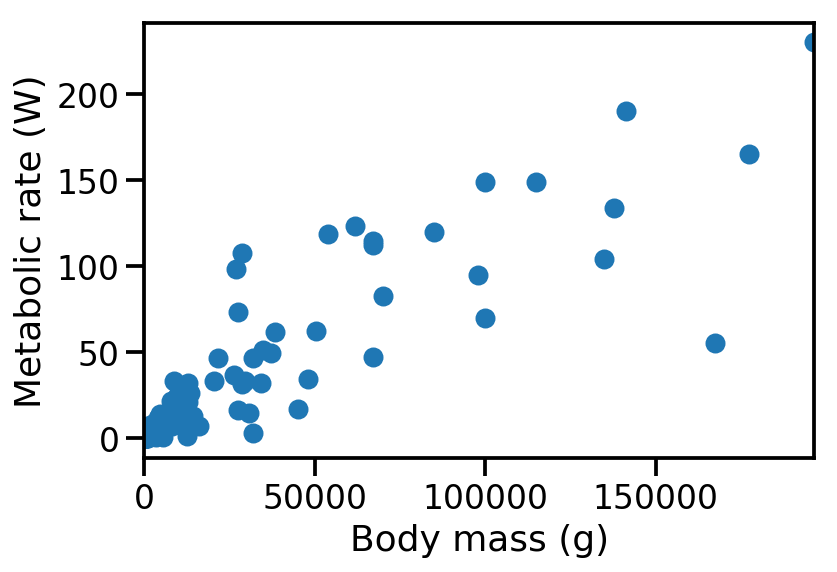If this plot looks funny, you are probably using Pandas with version <0.22; the bug was reported and fixed in version 0.22.

From this plot it seems that

1. there is a correlation between body mass and metabolic rate, and
2. there are many small animals (less than 30 kg) and not many medium animals (between 50 and 300 kg).

Before we continue, I prefer to have mass in kg, let's add a new column:

In :
data['Body mass (kg)'] = data['Body mass (g)'] / 1000


Next, let's check how many records do we have for each Class (as in the taxonomic unit):

In :
class_counts = data['Class'].value_counts()
print(class_counts)

Mammalia    417
Aves        171
Amphibia     18
Reptilia     16
Name: Class, dtype: int64

In :
class_counts.plot(kind='bar')
plt.ylabel('Num. of species');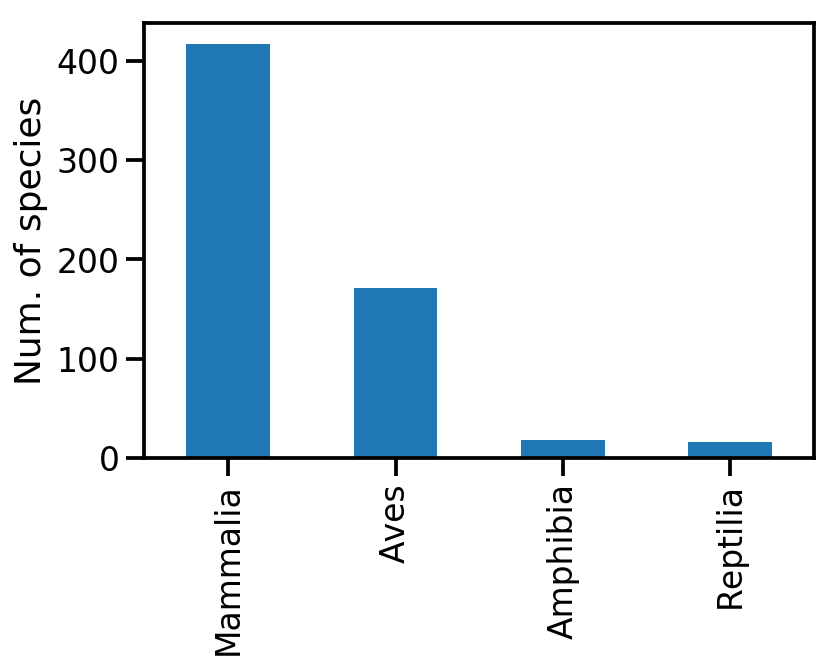So we have lots of mammals and birds, and a few reptiles and amphibians. This is important as amphibian and reptiles could have a different replationship between mass and metabolism because they are cold blooded.

## Exercise 1¶

1) Check how many reptiles are in this dataset, and how many of them are of the genus Python.

In :


In :
print("# of reptiles: ", reptiles)
print("# of pythons: ", pythons)

# of reptiles:  16
# of pythons:  2


2) Plot the number of species in each amphibian genus - use value_counts as above.

In :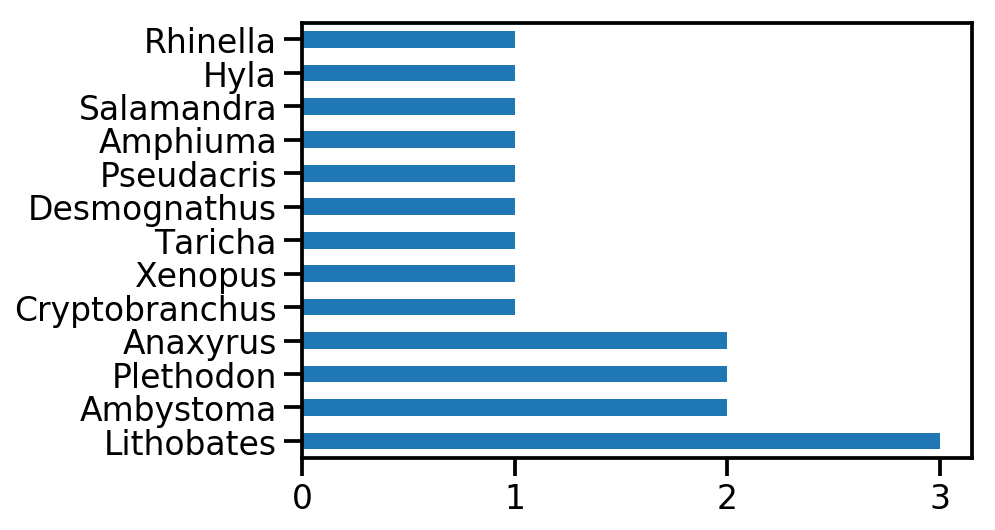# Seaborn¶

Let's do a simple linear regression plot; but let's do it in separate for each Class. We can do this kind of thing with Matplotlib and SciPy, but a very good tool for statistical visualizations is Seaborn.

Seaborn adds on top of Pandas a set of sophisticated statistical visualizations, similar to ggplot2 for R.

In :
import seaborn as sns
sns.set_context("talk")

In :
sns.lmplot(
x='Body mass (kg)',
y='Metabolic rate (W)',
hue='Class',
data=data,
ci=False,
);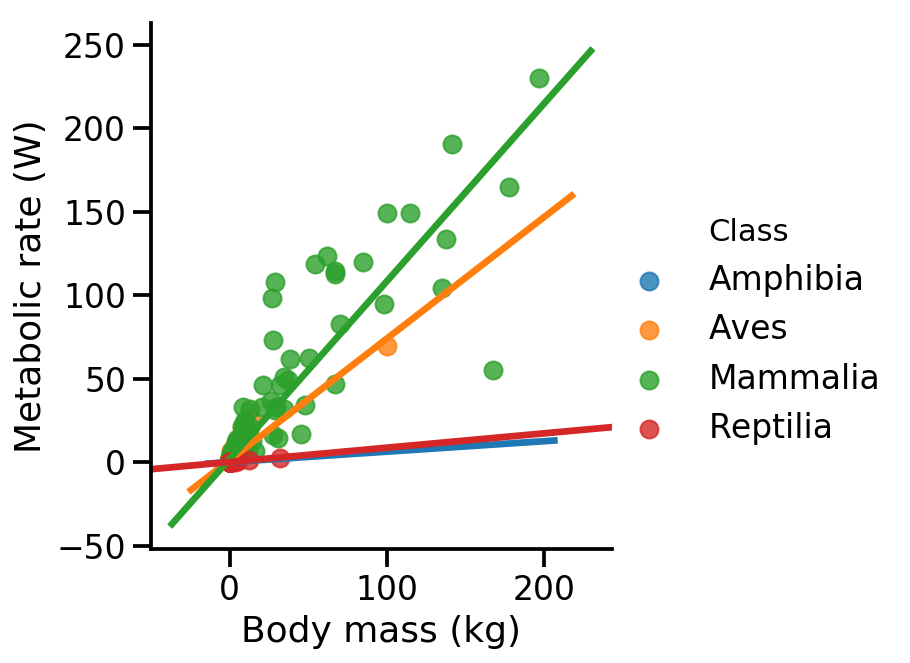• hue means color, but it also causes seaborn to fit a different linear model to each of the Classes.
• ci controls the confidence intervals. I chose False, but setting it to True will show them.

We can see that mammals and birds have a clear correlation between size and metabolism and that it extends over a nice range of mass, so let's stick to mammals; next up we will see which orders of mammals we have.

In :
mammalia = data[data['Class']=='Mammalia']
order_counts = mammalia['Order'].value_counts()
ax = order_counts.plot.barh()
ax.set(
xlabel='Num. of species',
ylabel='Mammalia order'
)
ax.figure.set_figheight(7)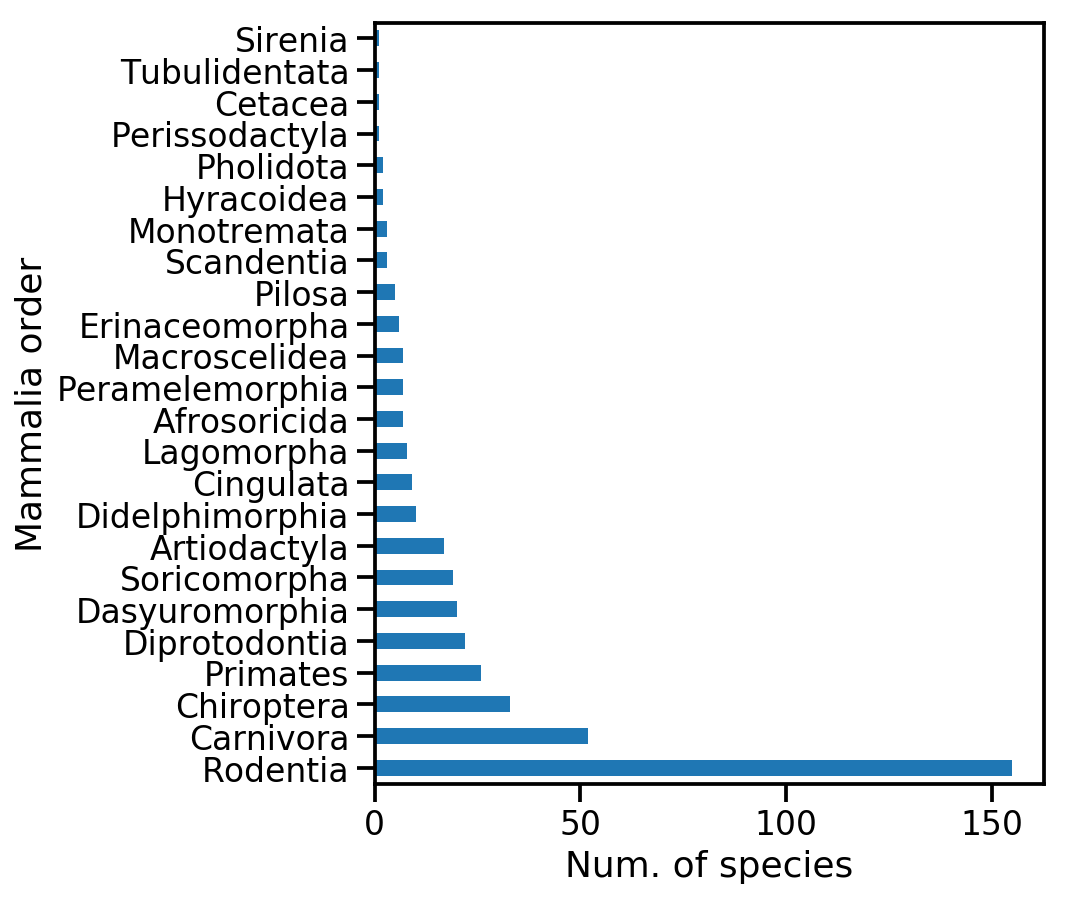You see we have alot of rodents and carnivores, but also a good number of bats (Chiroptera) and primates.

Let's continue with orders that have at least 20 species - this also includes some cool marsupials like Kangaroo, Koala and Taz (Diprotodontia and Dasyuromorphia)

In :
orders = order_counts[order_counts >= 20]
print(orders)
abund_mammalia = mammalia[mammalia['Order'].isin(orders.index)]

Rodentia          155
Carnivora          52
Chiroptera         33
Primates           26
Diprotodontia      22
Dasyuromorphia     20
Name: Order, dtype: int64

In :
sns.lmplot(
x='Body mass (kg)',
y='Metabolic rate (W)',
hue='Order',
data=abund_mammalia,
ci=False,
height=8,
aspect=1.3,
line_kws={'lw':2, 'ls':'--'},
scatter_kws={'s':50, 'alpha':0.5}
);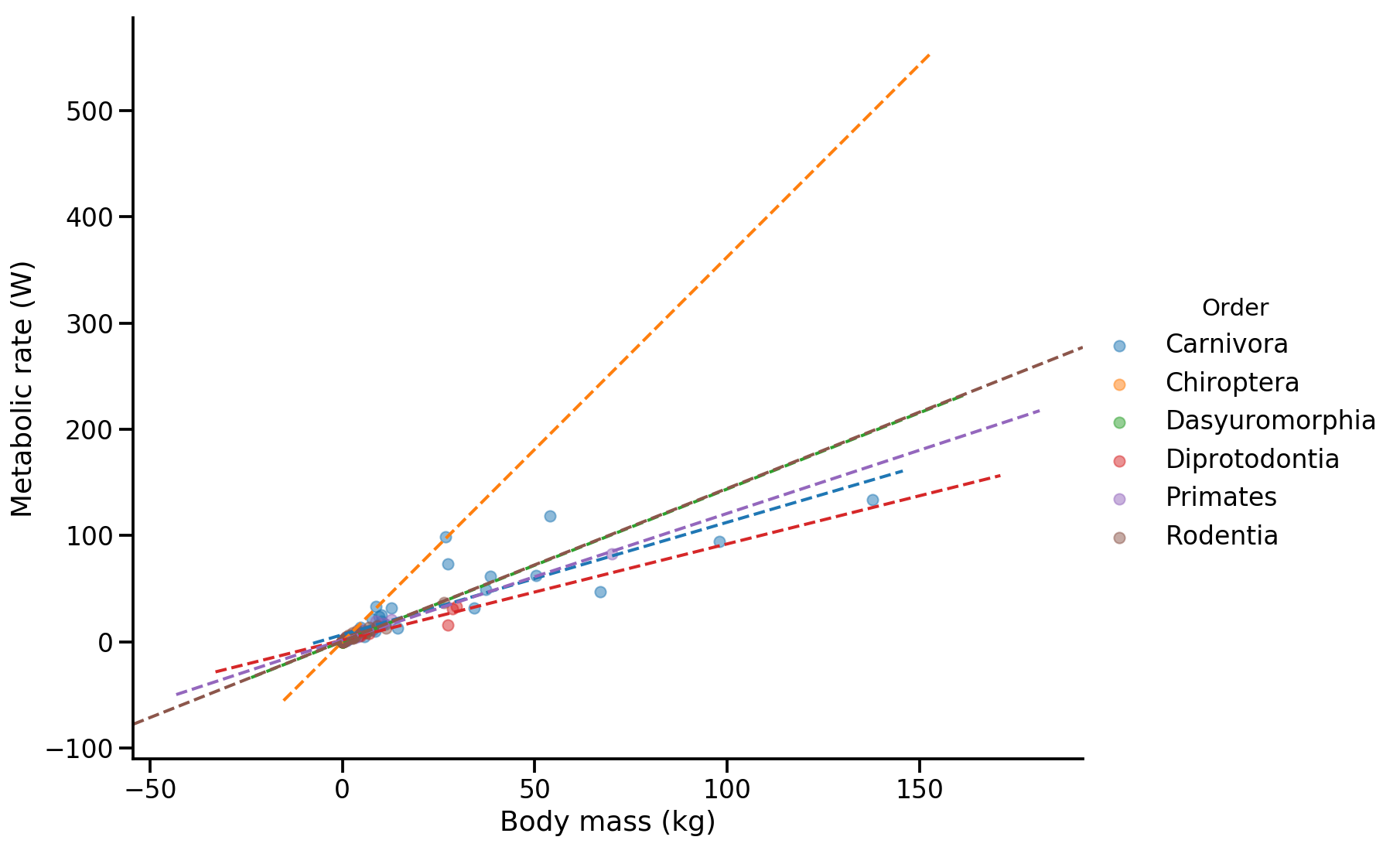Because there is alot of data here I made the lines thinner - this can be done by giving matplotlib keywords as a dictionary to the argument line_kws - and I made the markers bigger but with alpha (transperancy) 0.5 using the scatter_kws argument.

Still ,there's too much data, and part of the problem is that some orders are large (e.g. primates) and some are small (e.g. rodents).

Let's plot a separate regression plot for each order. We do this using the col and row arguments of lmplot, but in general this can be done for any plot using seaborn's FacetGrid function.

In :
sns.lmplot(
x='Body mass (kg)',
y='Metabolic rate (W)',
data=abund_mammalia,
hue='Order',
col='Order',
col_wrap=3,
ci=None,
scatter_kws={'s':40},
sharex=False,
sharey=False
);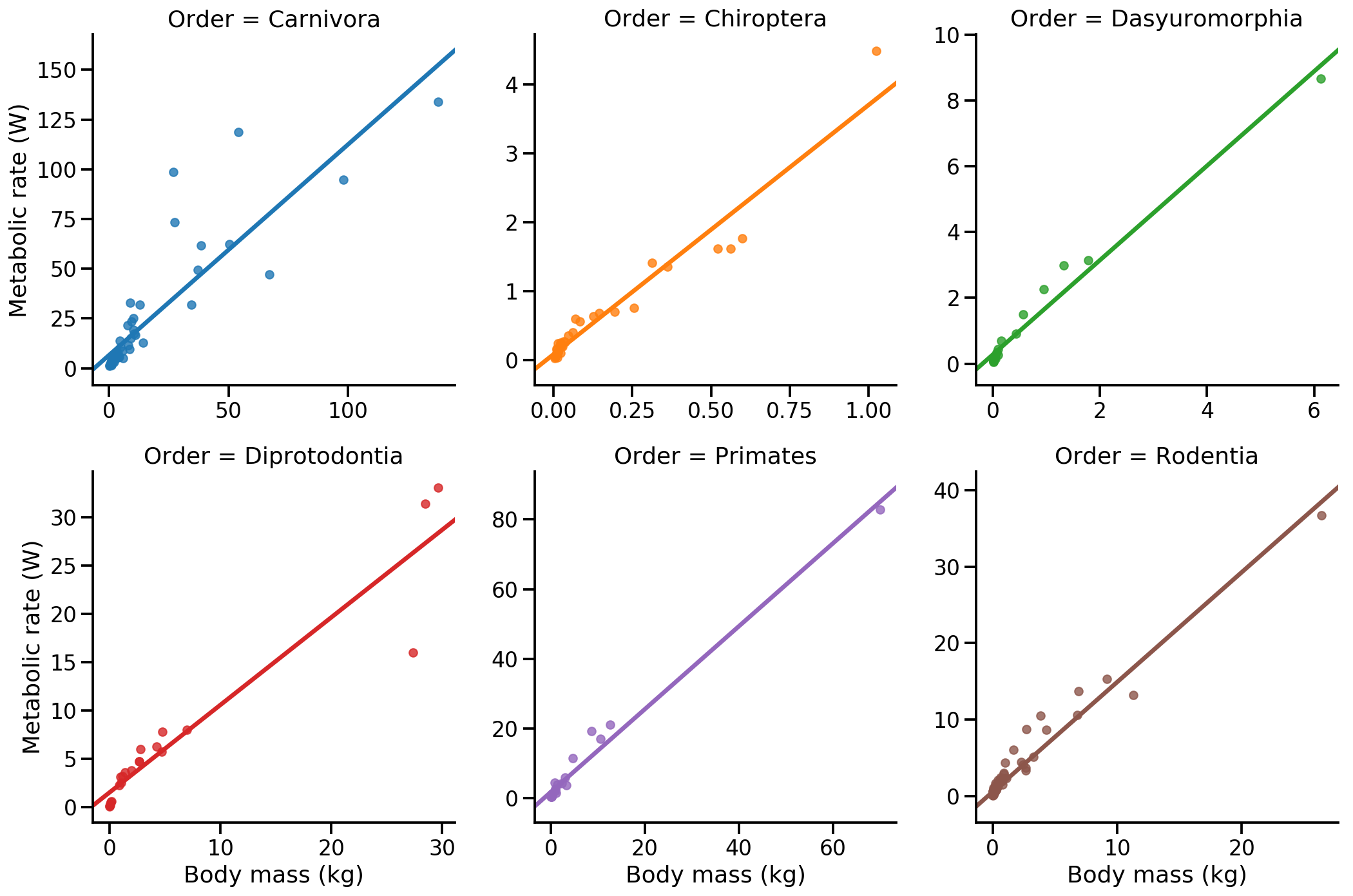We used the sharex=False and sharey=False arguments so that each Order will have a different axis range and so the data is will spread nicely. Last but not least, let's have a closer look at the corelation between mass and metabolism in primates. We will do a joint plot which will give us the pearson correlation and the distribution of each parameter.

You can disregard the warning, it appears because seaborn uses a deprecated keyword argument of matplotlib.

In :
primates = mammalia[mammalia.Order == 'Primates']
print(' | '. join(sorted(primates["Common name"])))
sns.jointplot(
x='Body mass (kg)',
y='Metabolic rate (W)',
data=primates,
kind='reg',
height=8
);

Blue monkey | Brown lemur | Calabar angwantibo | Demidoff's galago | Fat-tailed dwarf lemur | Geoffroy's tamarin | Greater galago | Guereza | Hamadryas baboon | Human | Mantled howler monkey | Northern night monkey | Patas monkey | Philippine tarsier | Potto | Pygmy marmoset | Senegal galago | Slender loris | Slow loris | Small-eared galago | South African galago | South American squirrel monkey | Spectral tarsier | Verreaux's sifaka | Western needle-clawed galago | White-tufted-ear marmoset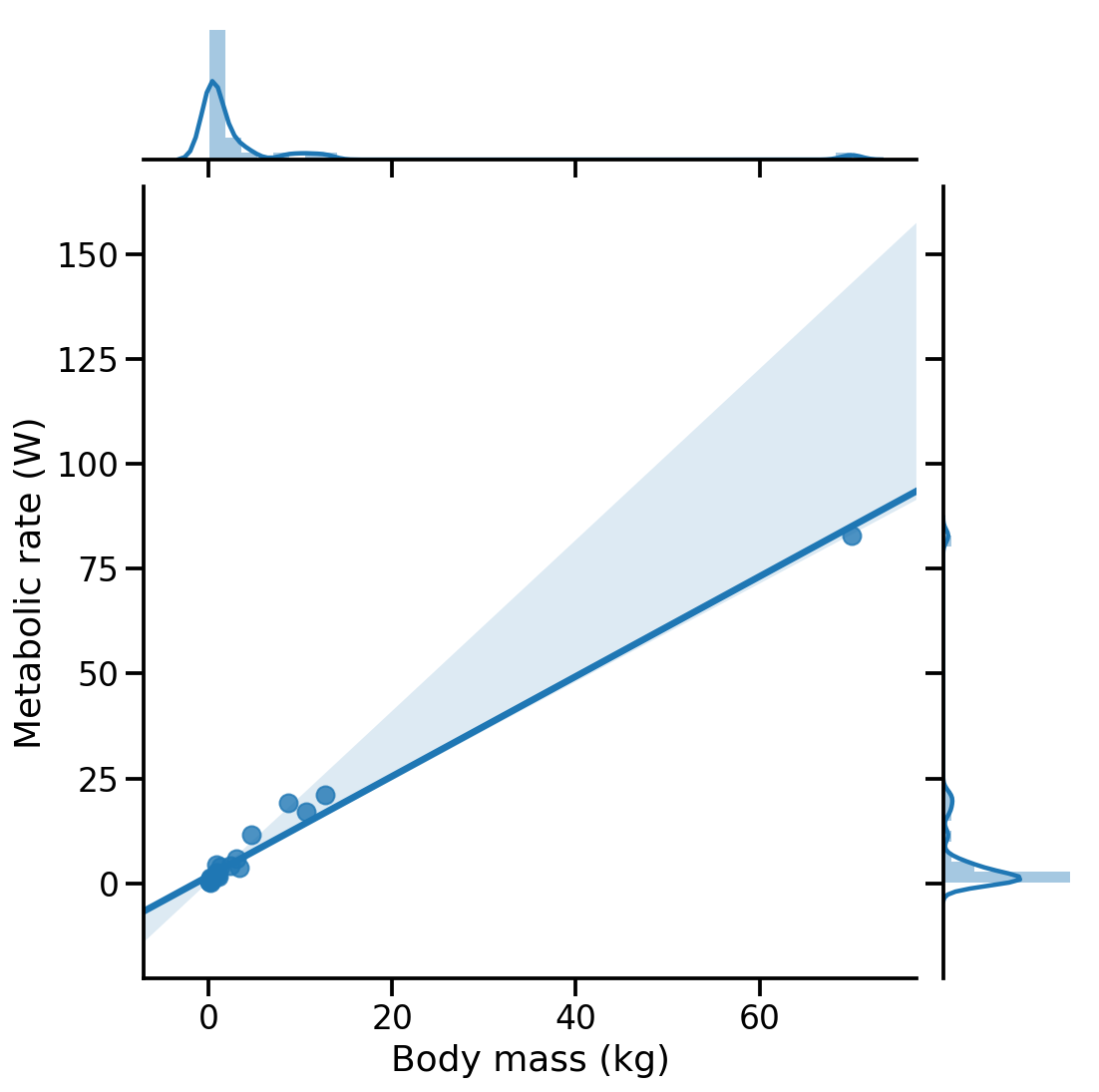# Colophon¶

This notebook was written by Yoav Ram and is part of the Data Science with Python workshops.

The notebook was written using Python 3.7. Dependencies listed in environment.yml.